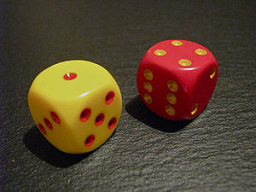# Probability 73054

We roll six dice. What is the probability that:
a) a six falls twice
b) six falls four times

p1 =  0.2009
p2 =  0.008

### Step-by-step explanation:Did you find an error or inaccuracy? Feel free to write us. Thank you!

Tips for related online calculators
Looking for a statistical calculator?
Would you like to compute the count of combinations?